# Physics - Conservation of Angular Momentum

see rotations theory

The angular momentum is the linear momentum times its distance from the axis of rotation.

When working out angular momentum its no use just summing the angular momentum's around the individual centres-of-mass of each body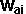*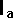+*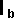=*+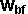*where:= initial angular speed of body A= initial angular speed of body B= final angular speed of body A= final angular speed of body B= second moment of mass about c-of-m of A= second moment of mass about c-of-m of B### Example 1

Imagine two bodies A and B of the same shape and mass. A is initially at rest, B is traveling toward A with no initial spin. So since they both started with with zero spin we might expect them to end up with equal and opposite spin.However that is not the case because A is hit in line with its own c-of-m so it gets no rotational component, however object B is not hit in line with its c-of-m so it will get a spin component.Therefore it is the angular momentum about the c-of-m OF THE WHOLE SYSTEM that is conserved?

I think this means that if, the point of impact is different from whole system c-of-m, then we need an extra term to include this extra rotational component about the system c-of-m.

But does this work? Consider another example:

### Example 2Two bodies with the same shape and mass, passing each other with equal and opposite speed and offset by distance r. Therefore the centre of mass of the whole system is stationary in the middle. Since the bodies are moving away, the moment of mass is increasing and the angle of rotation about the c-of-m is changing. But is the overall angular momentum remaining constant?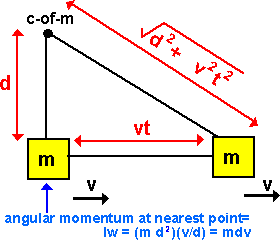From the above diagram the angular momentum at the nearest point to the c-of-m = m d vFrom the above after time t the angular momentum is still m d v

So angular momentum is conserved.

Thanks to Leo Wibberly for sending me this solution.

 metadata block see also: Correspondence about this page Book Shop - Further reading. Where I can, I have put links to Amazon for books that are relevant to the subject, click on the appropriate country flag to get more details of the book or to buy it from them.Physics for Game Developers - Assumes a knowledge of vectors, Matrix and trigonometry (the book has a one page introduction to quatnions). The book introduces Newtons laws but it does assume a basic knowledge physics. It covers Kinematics, Force, Kinetics, Collision (detection), Projectiles, Aircraft, Ships, Hovercraft, Cars, Real-time, 2D rigid body, Collision Response, Rigid body rotation, 3D rigid body, multiple bodies in 3D and particles. (I cant find a general formula for collision response which combines linear and rotation, but there may be something in the code included?). If you don't have the prerequisite knowledge of Matrices etc. you may want to get the Mathematics for 3D Game programming book first.Classical Dynamics of Particles and Systems - I have not reviewed this book, so I would be interested to hear if it is useful? I would also be interested to hear if there are any other books that may be of interest to readers of this page.Engineering Mechanics Vol 2: Dynamics - Gives theory for rigid dynamics, aims to allow prediction of effects of force and motion. Includes rotating frame of reference. Lots of colour diagrams, I guess its college / University level. Commercial Software Shop Where I can, I have put links to Amazon for commercial software, not directly related to the software project, but related to the subject being discussed, click on the appropriate country flag to get more details of the software or to buy it from them.

This site may have errors. Don't use for critical systems.